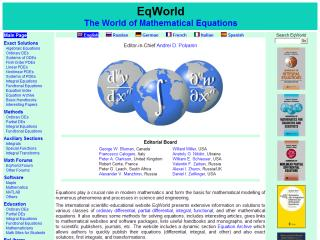## Tuesday, April 21, 2009

### Mathematical Equations Bible - Handbook For FEA Engineers

Hello viewers, if you have read my previous article about Engineering Mathematics and today's topic is related to Math as well. Lets see advance Math which are effectively used in Engineering. The site which we are going to see now is full of mathematical equations like Partial Differential Equations,Algebraic Equations,Integral Transforms etc.

Equations play a crucial role in modern mathematics and form the basis for mathematical modelling of numerous phenomena and processes in science and engineering.

The international scientific-educational website EqWorld presents extensive information on solutions to various classes of ordinary differential, partial differential,integral, functional, and other mathematical equations. It also outlines some methods for solving equations, includes interesting articles, gives links to mathematical website's and software packages, lists useful handbooks and monographs, and refers to scientific publishers, journals, etc. The website includes a dynamic section Equation Archive which allows authors to quickly publish their equations (differential, integral, and other) and also exact solutions, first integrals, and transformations.The EqWorld website is intended for researchers, university teachers, engineers, and students all over the world. It contains about 2000 webpages and is visited by over 2500 users a day (coming from 200 countries worldwide). All resources presented on this site are free to its users.

I believe this site will help many engineering students as well as professionals that too FEA engineers who are looking at numerical methods. The story still continues, the exciting part of this site is it has got software section where you can see

Mathematica: A powerful symbolic computation system by Wolfram Research

Maple: An alternative symbolic computation system by Waterloo Maple, Inc.

MATLAB: A popular system for numerical solution of differential equations and data visualization by The MathWorks, Inc.

The DOE ACTS Collection: The Advanced CompuTational Software (ACTS) Collection is a set of software tools for computation sciences.

Dictionary of Algorithms and Data Structures (NIST): The dictionary of algorithms, algorithmic techniques, data structures, archetypical problems, and related definitions

Mathcom: Partial Differential Equations and Finite Element Modeling

Courtesy : Eqworld.com

#### 1 Comment:

Anonymous said...

Good Job.. Like to subscribe your posts :)